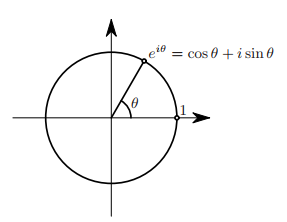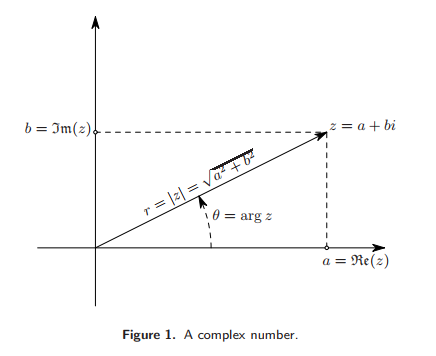# Number System - Imaginary Number

An imaginary number i is one solution to $x^2 = -1$ and is a part of the definition of a complex number

$$i = \sqrt { -1 }$$

It was invented because formulas sometimes required the manipulation of square roots of negative numbers.

Numbers such as i, -i, 3i, 2.17i are called imaginary number.

## Example

• Problem: $x^2 = -9$
• Solution: x = 3i

## Documentation / Reference

Recommended PagesMathematics - Complex Exponential (Euler's formula)

Euler's formula provides a powerful connection between analysis and trigonometry, and provides an interpretation of the sine and cosine functions (the sinusoidal functions) as weighted sums of the exponential...
Number System (Classification|Type)

Numbers are classified according to how they are represented or according to the properties that they have. They have what's called a type. For systems for expressing numbers, see Numeral system. ...Number System - Complex Number -

A real number plus an imaginary number is a complex number. Complex numbers are a number system. A complex number has a real part and an imaginary part. where: i is an imaginary number Complex...
Number System - Real Number (Scalar) -

A real number is a value that represents a quantity along a line. They have been called real to distinguish them from imaginary number. imaginary roots of polynomials The real numbers include all...